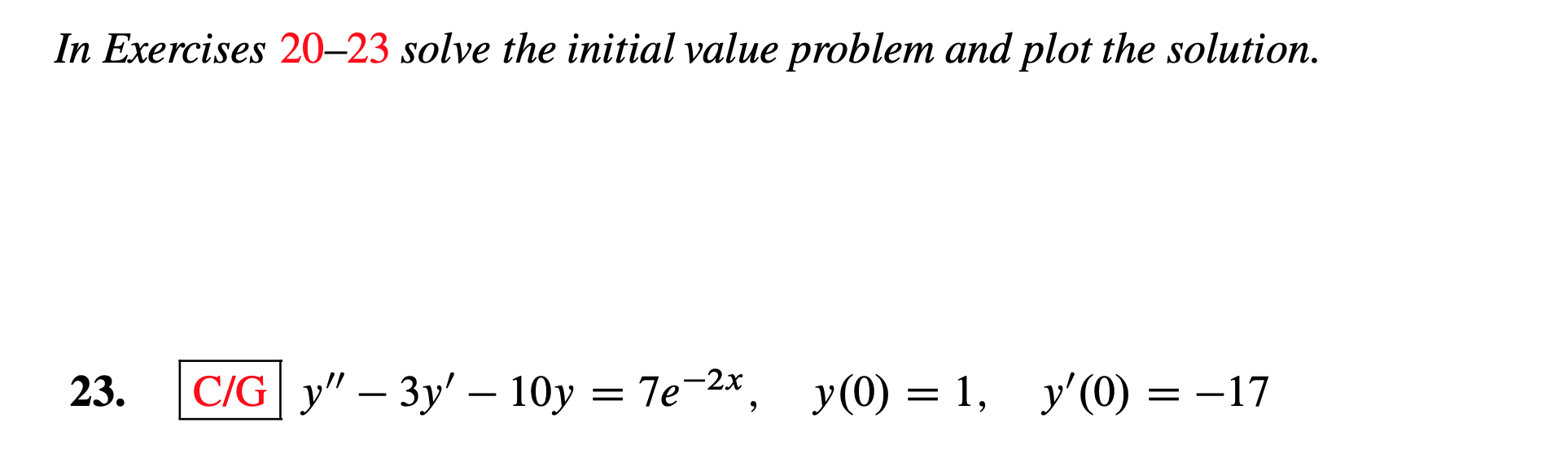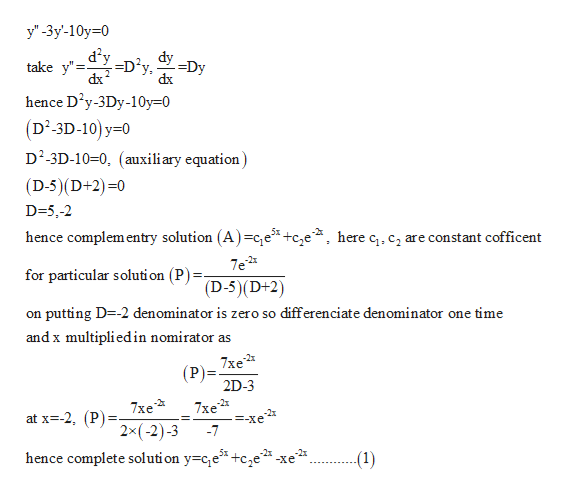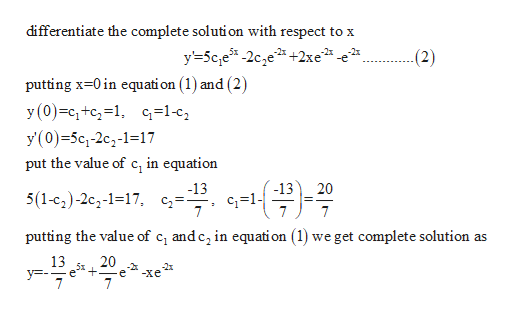# In Exercises 20-23 solve the initial value problem and plot the solution%3 Те 2*, у(0) — 1, у'(0) — —CIG y" - Зу' — 10у-2x23=-17= Te

Question
3 viewshelp_outlineImage TranscriptioncloseIn Exercises 20-23 solve the initial value problem and plot the solution %3 Те 2*, у(0) — 1, у'(0) — — CIG y" - Зу' — 10у -2x 23 =-17 = Te fullscreen
check_circle

Step 1

Given equation is nonhomogenious linear differential equation solution for this type of equation complementry (A) and particular(P) solution should be find and it is written as y=A+P

Given differential equation

Step 2

For complementry solution take homogenious part ashelp_outlineImage Transcriptioncloseу' -Зу-10y30 dy dy take y" D?y dx -=Dy dx hence D2y-3Dy-10y 0 (D'-3D-10) у-0 D2-3D-10-0, (auxili ary equation) (D-5)(D+2) 0 D-5,-2 hence complementry solution (A)=ce +cje^, here c, c2 are constant cofficent 7e2 for particular solution (P) (D-5)(D+2) on putting D -2 denominator is zero so differenciate denominator one time and x multipliedin nomirator as 7xe (P 2D-3 7xe 2x(-2)-3 7xe 2 -Xe -7 at x--2, (P) +c,e%* -xe2 hence complete soluti on y c,e (1) fullscreen
Step 3

take first defferentiation of o...help_outlineImage Transcriptionclosedifferentiate the complete solution with respect to x y'5ce -2c,e2 +2xe*. -2x -e (2) putting x-0 in equation (1) and (2) y (0) c1+c2=1. cq=1-c2 y'(0)-5c1-2c2-1-17 in equation put the value of -13 -13 20 5(1-c2)-2c2-1-17, c* 1 7 7 putting the value of c and c2 in equati on (1) we get complete solution as 13 y- 7 20 -2x -Xe 7 fullscreen

### Want to see the full answer?

See Solution

#### Want to see this answer and more?

Solutions are written by subject experts who are available 24/7. Questions are typically answered within 1 hour.*

See Solution
*Response times may vary by subject and question.
Tagged in

### Calculus